Industrial Data Science
in C# and .NET:
Simple. Fast. Reliable.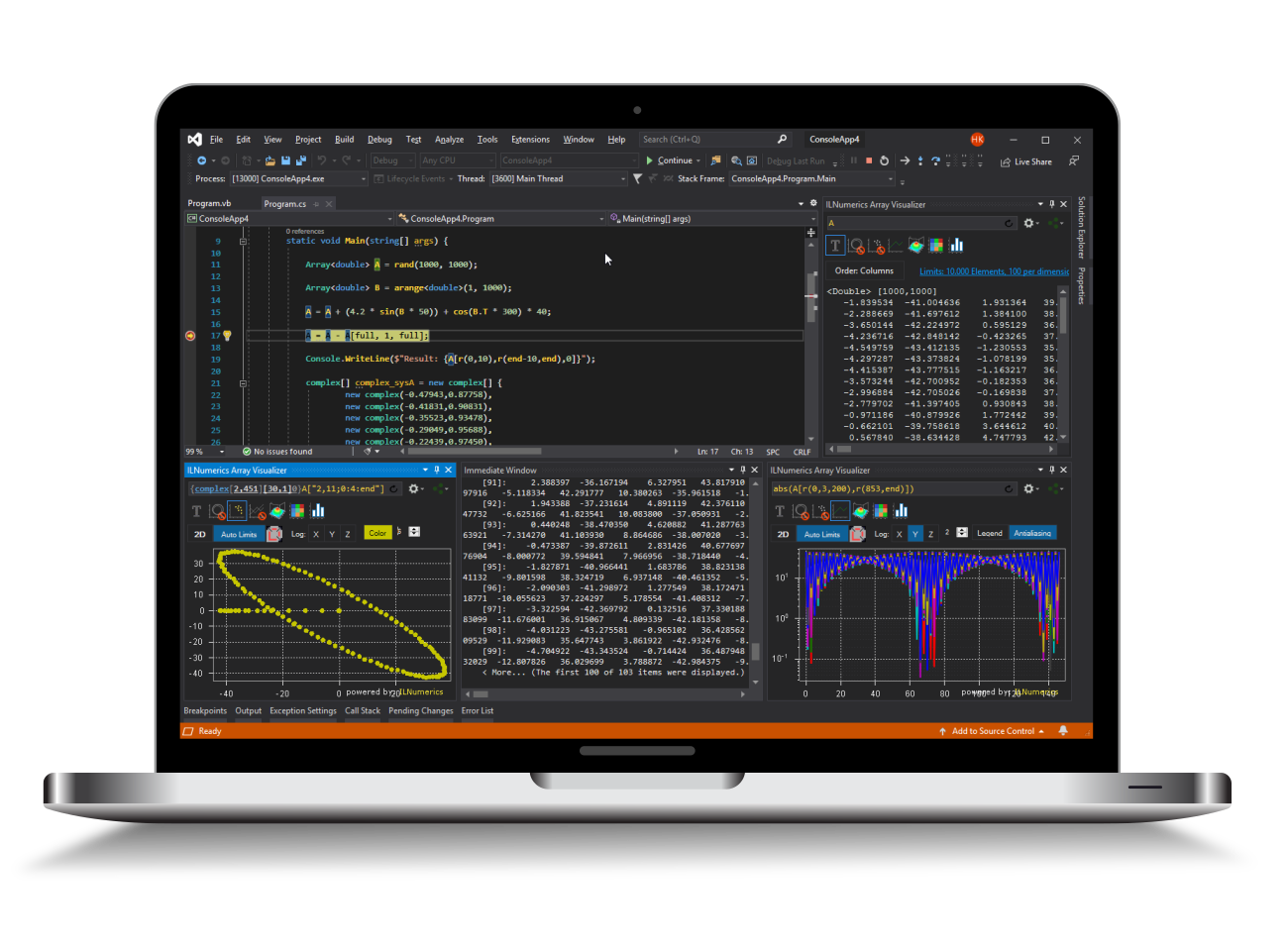ILNumerics - Technical Computing

Modern High Performance Tools for Technical

Computing and Visualization in Industry and Science

tgt

# Kriging Interpolation in .NET (C#, Visual Basic)

Kriging is a popular interpolation and regression method, originally applied in geostatistics. It is now increasingly used for general 1D, 2D and n-dimensional interpolation problems, scattered data interpolation, curve fitting in N dimensions, multi-valued problems and many more. In fact, kriging is the only interpolation method in ILNumerics Interpolation Toolbox, which is applicable in all interpolation scenarios.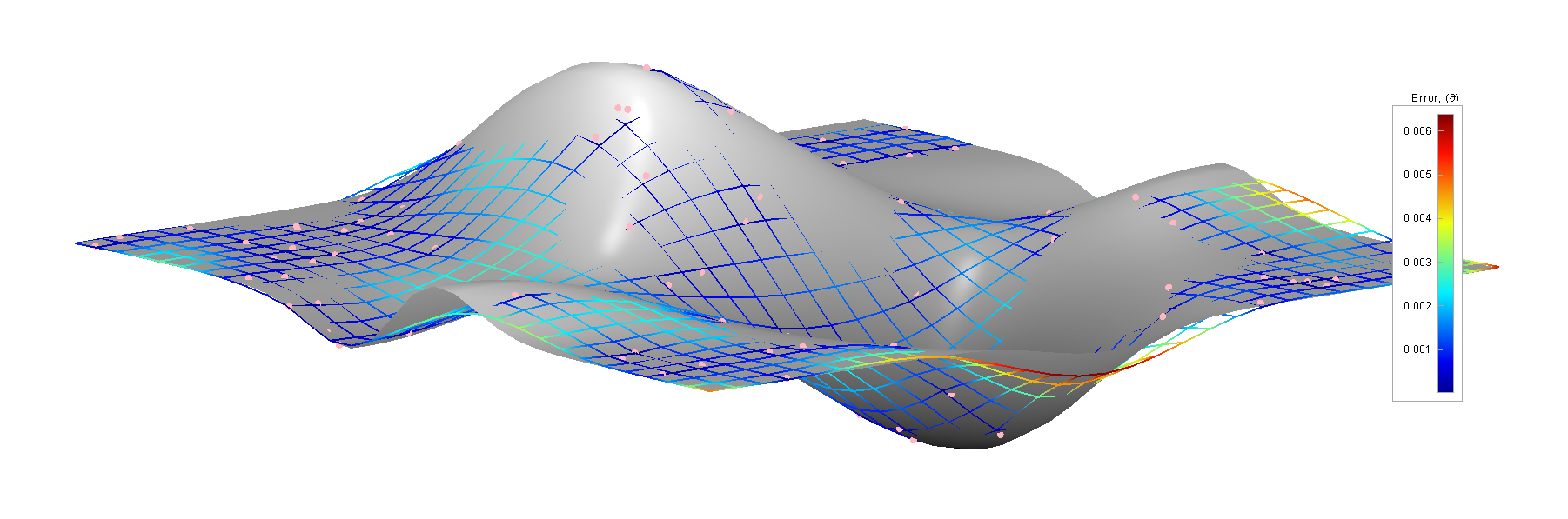## ILNumerics Kriging Interpolation Overview

Kriging learns statistical properties from a set of provided sample values. Values for new points are interpolated based on their distance to the original points by taking the topology of the sample set and the estimated variance of an assumed underlying random process into account. Kriging is a gaussian process and it predicts the best unbiased interpolated values possible. If necessary, the properties of the underlying statistical model can be widely adjusted.

Kriging interpolation is often favorable over other interpolation methods due to its capability to provide error estimates (variances) for interpolated values. Furthermore, Kriging brings interesting properties for scattered data interpolation: it limits the over-estimation often caused by clusters in the source data.

ILNumerics Interpolation Toolbox implements kriging interpolation with the following properties:

• Interpolation for n-dimensional, multi-valued data sets,
• Parameterless automatic adaption of variogram properties, or
• Arbitrary optional custom variogram functions,
• Sanity checks for the input parameters,
• Automatic removal of redundant values,
• Optimized for minimum memory usage and maximum performance.
• Values obtained by kriging interpolation will always pass through the original support points.

Two common APIs are provided for kriging (as for all interpolation techniques within ILNumerics). The static Interpolation.kriging() function is used for 1-time interpolation of data sets. Alternatively, interpolator objects can be created and reused for more efficient computations. In the following section we will demonstrate both options.

## 1D Kriging Interpolation

The following example takes 20 sample points from a function $f$ in $R$. The sample points are used with the Interpolation.kriging() function. The following code is part of a larger example which can be retrieved from the examples section.

The work is done in the ILNumerics.Toolboxes.Interpolation.kriging(X,Y) function. In the example, we create interpolated values for all positions $X$ based on 20 randomly sampled values from $f$. These samples are plotted as gray dots. The original function is shown as a black line. And the interpolated values are shown as red line. The result looks similar to the following plot: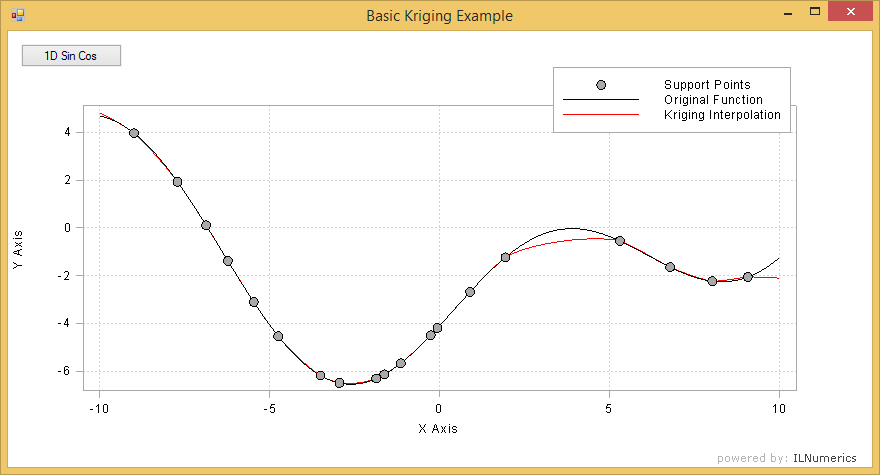Note how the interpolation result reflects the common experience with any interpolation method: interpolations works resonably well for regions which are sufficiently supported by given sample points. As we move further away from known values the result more and more significantly deviates from the original function. This is easy to determine as long as the original function is known. However, in practice this is mostly not the case. Luckily, kriging gives you some unique options to derive some confidence for your interpolation results. This is shown further down at the end of this article.

## 2D Kriging Interpolation

Just like for the 1-dimensional case kriging interpolation can be used on multi-dimensional data. The creation of the interpolator object and the application of new sample data does not differ from the 1D case. The source data X provided to the kriging() function is expected to be layed out as common matrix: individual data points are stored as columns of length d. Note that if the source data would expose some geometrical relation to each other (let's say: the data could be ordered as a grid) that order would not be taken into account. Kriging deals with scattered data and (re-)computes such ordered relation between individual data points in a very different manner.

Example: Two-dimensional kriging interpolation.

The dimension length of new data points given to the kriging interpolator must correspond with the dimension length d of the source data. In the above example two dimensional data points are used for kriging interpolation. The interpolator is created based on scattered data over a range of [-3, -3] -> [3, 3]. Roughly 100 scattered points are sampled at random positions and serve as the only data source for the kriging interpolator. With the resulting interpolator a regular grid of 900 points is evaluated and interpolated values are computed for the grid points. The interpolated grid is shown as colored wireframe in the plot.

Note how the underlying function, while exposing strong curvature, is interpolated closely with only a small error at those regions of spare supporting sample points.Here is another image of a potential result: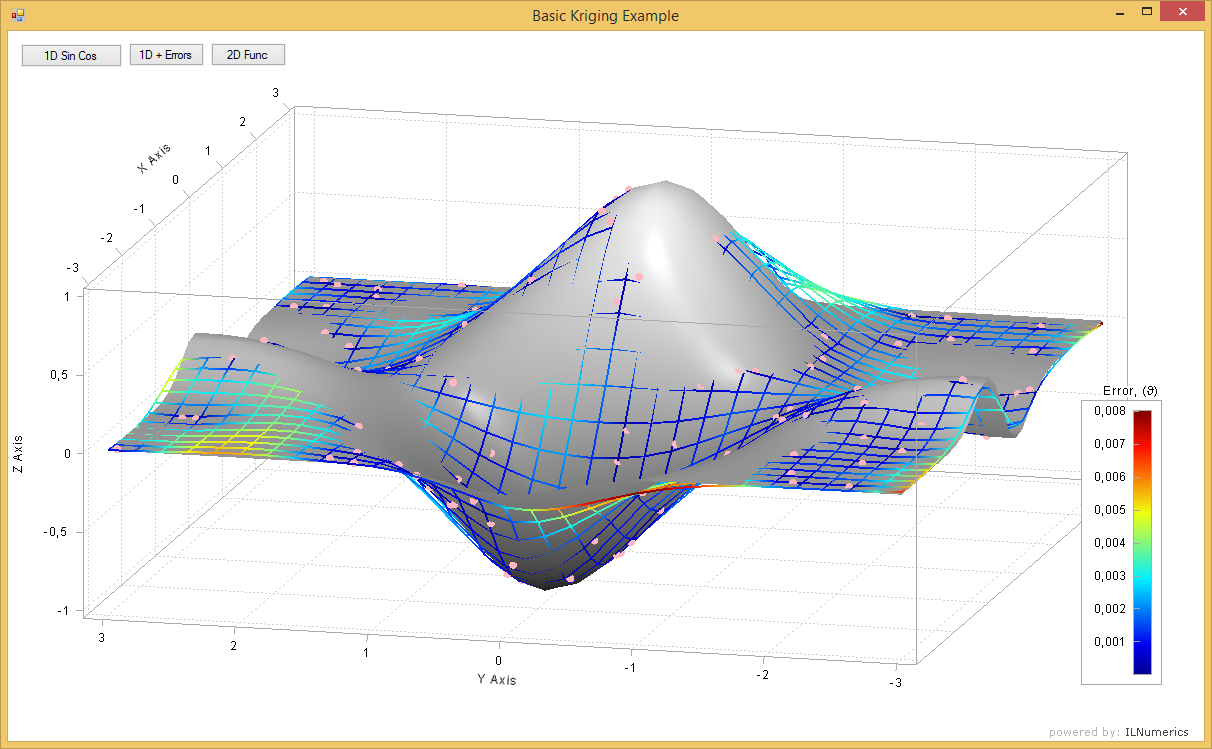The complete, runnable example is found in the examples section or via the WebGL renderer in the web code component above.

## N-dimensional Kriging Interpolation

N-dimensional data is applied to the kriging interpolation in the very same way as 2-dimensional interpolation. The data are expected as columns in the positions matrix X. Please refer to the 2D case shown above.

## Custom Variogram Functions

Kriging computes new values for new points based on the topology of original points and the change in the variance according to the distance to those original points. The variance of the underlying random variable (see: gaussian process) is expected to vary only with the distance, or lag $h$ – not by the actual position of the point. Therefore, the random field is expected to be stationary.

The function used for computing the variation of the variance in dependence on $h$ is called a variogram. Several variogram types exist for specific areas of interest: spherical, exponential and gaussian variogram functions typically require certain additional parameters which in practice need to be acquired empirically from measurements.

For interpolation, however, it is often sufficient to use a simple variogram function which gives an exponential decay of the variance for increasing lag. The default function implemented in ILNumerics Interpolation Toolbox is a power law function:

$$\theta(h) = \alpha h^\beta$$

The parameters $\alpha$ and $\beta$ are automatically determined from the data (positions and values) provided to the kriging function. If need arises, both parameters can be adjusted for a kriging interpolator object (see below):

When specific variogram parameters are available, ILNumerics Interpolation toolbox allows to implement a custom variogram function easily and use that for the kriging interpolation:

Here, we have used empirical variogram property data for the implementation of our custom variogram function. The result may looks similar to this: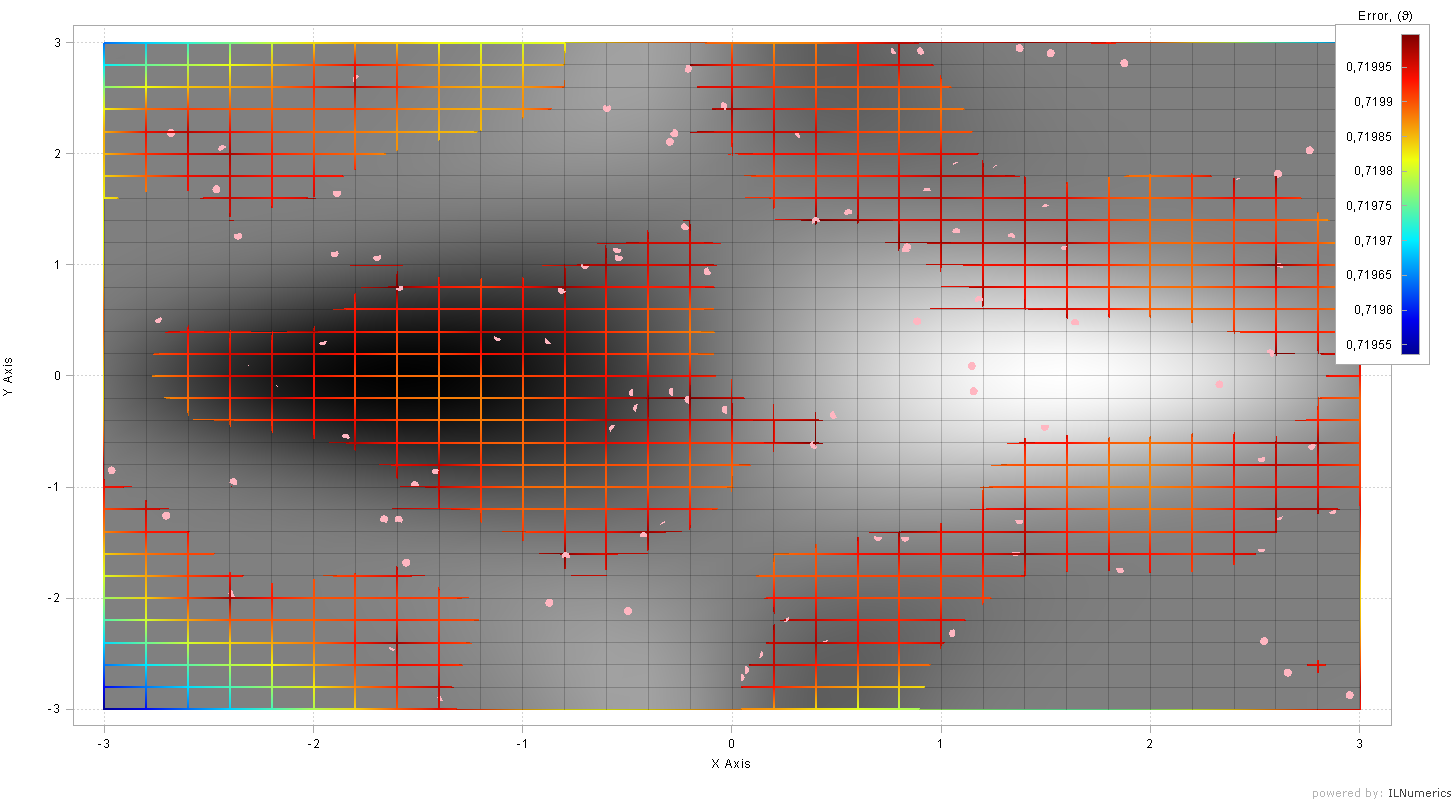Clearly, the choice of variogram function strongly influences the error (uncertainty) of the data retrieved. However, commonly the variogram function is of less importance to the general interpolation result. Even crude variogram functions can bring good interpolation results. As with all interpolation methods one should evaluate the result for specific requirements.

## Interpolator Object API

Kriging interpolation – as almost any interpolation techniques – involves a two step process:

1. First a set of sample points is used to create an interpolator object. Most computational effort is spent in this step.
2. The kriging interpolator is afterwards used to compute new interpolated values in a fast and efficient process.

The static Interpolation.kriging() function wraps these steps for your convenience. For large datasets and many repeated interpolations based on the same source data using the underlying interpolator objects directly can be profitable by saving repeated efforts for the creation of such interpolator objects.

Example: Using the kriging interpolator objects.

The interpolator object is used within a using block (C#, Using in Visual Basic). The object internally creates and stores intermediate data which are reused for subsequent interpolations. By utilizing the interpolator inside a using block we ensure that this data get released when no longer needed.

One can apply the interpolator object to new data points as often as necessary. The application (interpolation) for new values will be much faster than the initial creation of the interpolator. In terms of efforts: the creation needs $O(N^3)$, the interpolation of a new data point requires $O(N)$ effort, where $N$ is the number of data points V initially provided. (Similar numbers are valid in terms of memory requirements.)

Following kriging interpolator objects exist in the ILNumerics.Toolboxes namespace for the corresponding supported element types: KrigingInterpolatorDouble, KrigingInterpolatorSingle, KrigingInterpolatorComplex, and KrigingInterpolatorFComplex.

## Retrieving Error Information

Kriging offers the option to compute information about the error of interpolated values. The more we get away from known samples, the more the interpolation result becomes a guess, obviously. Nevertheless, in the case of kriging interpolation the result is always the most likely guess according to the known samples. Since every sample value in kriging is seen as the instance of a random process, the value itself is associated with the mean of the random variable. In difference to that the error corresponds to its variance.

Let's create another interpolation example! This time we compute not only new interpolated values for a set of given samples. We also compute error information for them.

The kriging interpolator is created in exactly the same way as before. We used another overload of the Apply function which provides the error information with every computed interpolated data point as an additional output parameter. Errors are returned as a vector. It holds a scalar value which corresponds to the expected variance at the position of each new value X.

In order to plot the error we add two more line plots to the graph. One showing the upper error range $E_u$ and another for the lower error range $E_l$.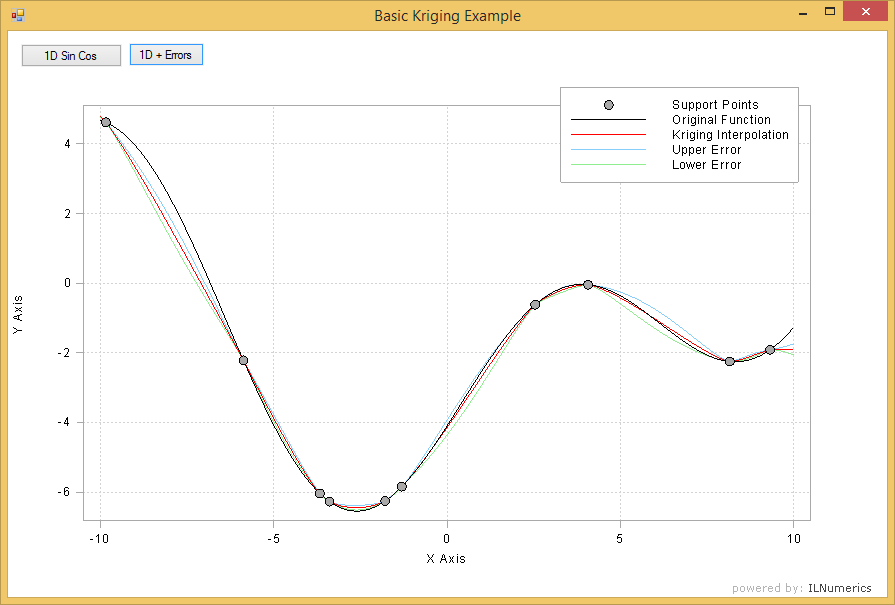Note how the error $err$ returned from Apply() determines the whole error range, i.e. the range above and below the interpolated values. Therefore, for plotting we added half of the error range to each side of the interpolated result.

Note further that the error does not allow any absolute quantization of the exact deviation of the interpolated values from the actual underlying function! This would obviously only be possible, if that function would be completely known. Instead, err values give a reasonable hint about the variance of the most likely (random) variable at that position.

As can be clearly seen from the image above, having error information does not protect one from misleading results. We intentionally chosed too few samples according to the true function. Consequently, for regions of high frequency (strong curvature) we see the risk of the interpolation values not matching the underlying function sufficiently. Let's take the region around the X value -7 as one example: here, the true function (black) does not even lay in the range provided by the upper and lower error range.

The only way to prevent from such deviations is to provide enough support points at the regions of interest, if possible: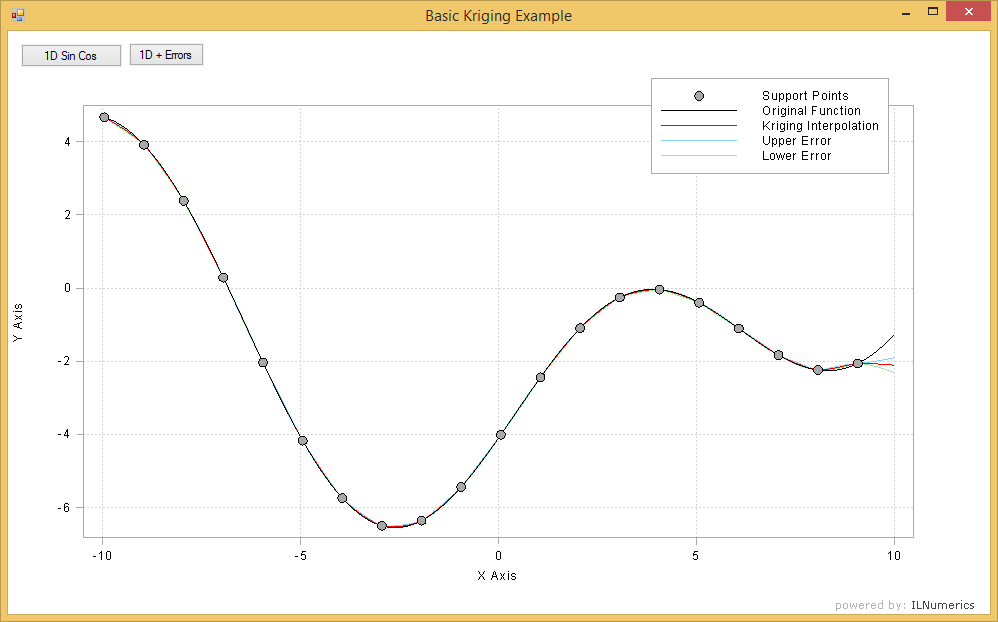Let's take another, closer look at the 2D kriging interpolation example from an error information-point of view. A number of 100 samples (red dots) are taken from a known function (gray surface) at random positions and used as source for kriging interpolation. The interpolated points (wireframe) are plotted over the true function. The error information acquired from the kriging interpolation is color coded on the wireframe.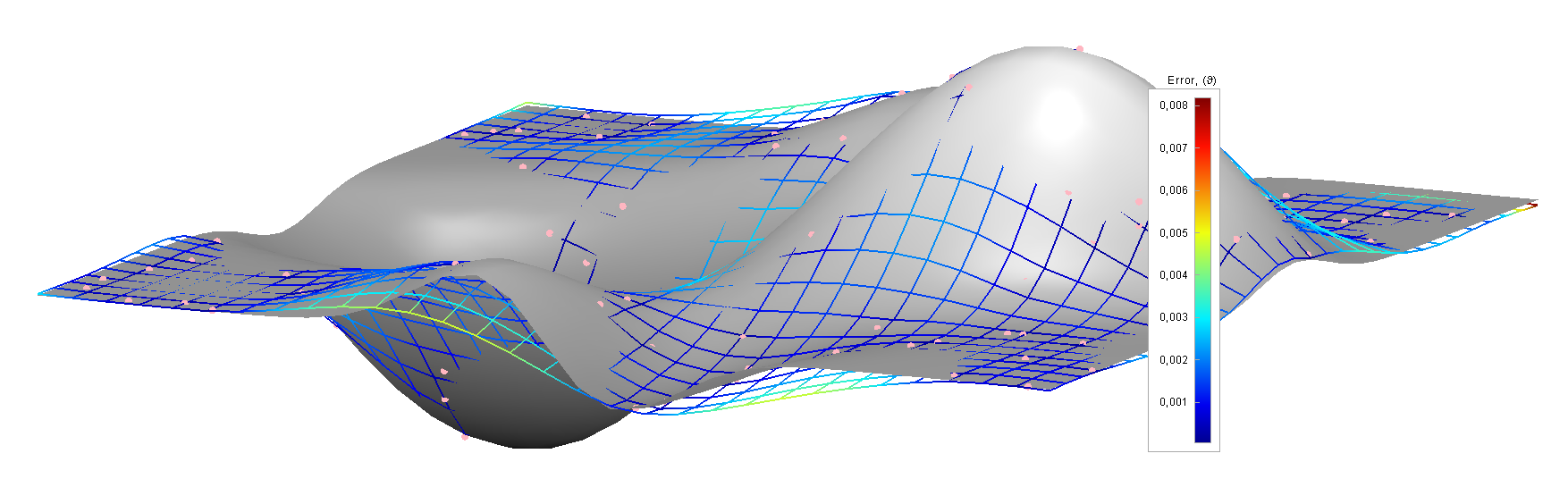As can be seen from the image, regions of higher uncertainty (variance, or "error") are marked with yellowish or red wireframe colors. Indeed for these regions the interpolated wireframe often shows a larger deviation from the true function (gray surface) than for blue regions of lower variance. However, there are also interpolated regions visisble which are marked with red wireframes but show a good match to the original function! So what the error information computed by kriging really means is: uncertainty. If the error is small we can trust the returned value more than for higher error values. It does not mean that for higher errors the values are necessarily wrong. We simply don't know.